Next: Generalized momenta Up: Lagrangian mechanics Previous: Generalized forces

# Lagrange's equation

The Cartesian equations of motion of our system take the form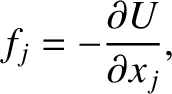(7.9)

for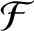, where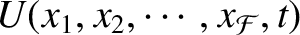are each equal to the mass of the first particle,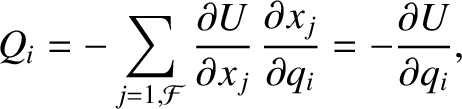are each equal to the mass of the second particle, and so forth. Furthermore, the kinetic energy of the system can be written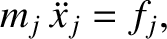(7.10)

Because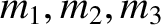, we can write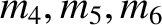(7.11)

for. Hence, it follows that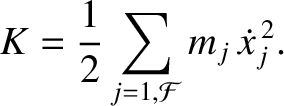. According to the preceding equation,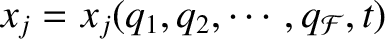(7.12)

where we are treating the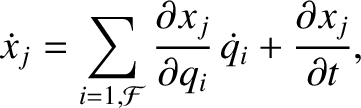and the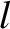as independent variables.

Multiplying Equation (7.12) by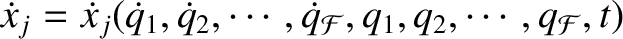, and then differentiating with respect to time, we obtain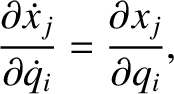(7.13)

Now,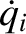(7.14)

Furthermore,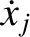(7.15)

and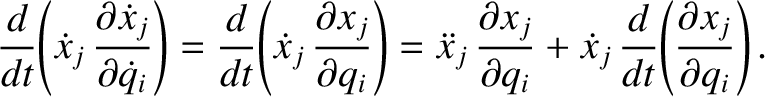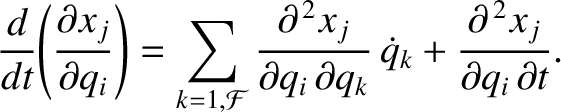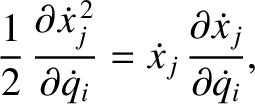(7.16)

where use has been made of Equation (7.14). Thus, it follows from Equations (7.13), (7.15), and (7.16) that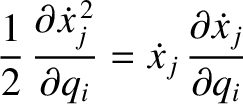(7.17)

Let us take Equation (7.17), multiply by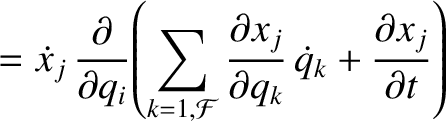, and then sum over all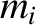. We obtain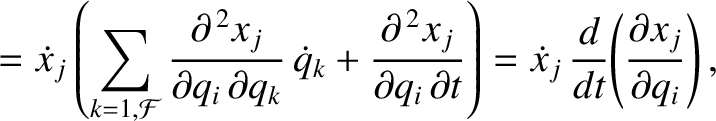(7.18)

where use has been made of Equations (7.9) and (7.10). Thus, it follows from Equation (7.6) that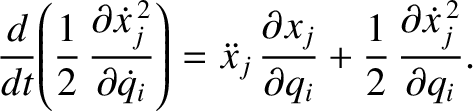(7.19)

Finally, making use of Equation (7.8), we get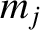(7.20)

It is helpful to introduce a function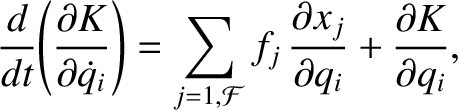, called the Lagrangian, which is defined as the difference between the kinetic and potential energies of the dynamical system under investigation: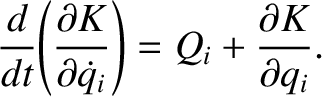(7.21)

Because the potential energy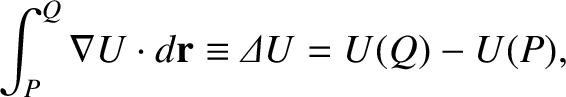is clearly independent of the, it follows from Equation (7.20) that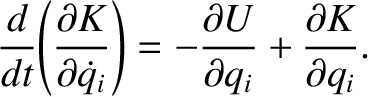(7.22)

for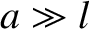. This equation is known as Lagrange's equation.

According to the preceding analysis, if we can express the kinetic and potential energies of our dynamical system solely in terms of our generalized coordinates and their time derivatives then we can immediately write down the equations of motion of the system, expressed in terms of the generalized coordinates, using Lagrange's equation, Equation (7.22). Unfortunately, this scheme only works for conservative systems.

As an example, consider a particle of massmoving in two dimensions in the central potential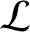. This is clearly a two-degree-of-freedom dynamical system. As described in Section 4.4, the particle's instantaneous position is most conveniently specified in terms of the plane polar coordinates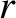and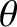. These are our two generalized coordinates. According to Equation (4.13), the square of the particle's velocity can be written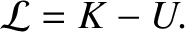(7.23)

Hence, the Lagrangian of the system takes the form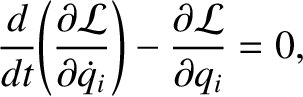(7.24)

Note that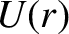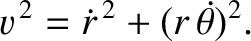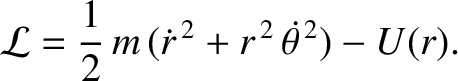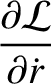(7.25)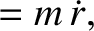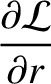and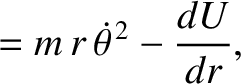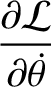(7.26)

Now, Lagrange's equation, Equation (7.22), yields the equations of motion,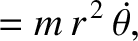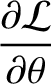(7.27) and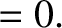(7.28)

Hence, we obtain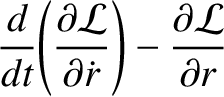(7.29) and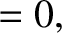(7.30)

or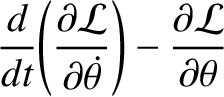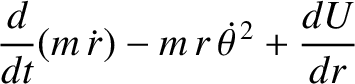(7.31) and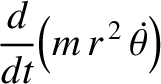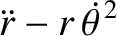(7.32)

where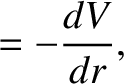, and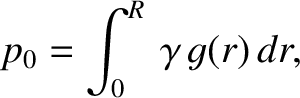is a constant. We recognize Equations (7.31) and (7.32) as the equations that we derived in Chapter 4 for motion in a central potential. The advantage of the Lagrangian method of deriving these equations is that we avoid having to express the acceleration in terms of the generalized coordinatesand.Next: Generalized momenta Up: Lagrangian mechanics Previous: Generalized forces
Richard Fitzpatrick 2016-03-31